##### 2. switch Statement :-

This is multi-branching statement. Syntax of this statement is as follows:

switch (expression/variable)
{ case value_1: statement -1;
break;
case value_2: statement -2;
break;
:
:
case value_n: statement -n;
break;
[ default: statement -m ]
}

Note: expression/variable should be integer or character type only. When the switch statement is executed, the expression/variable is evaluated and control is transferred directly to the statement whose case label value matches the value of expression/ variable. If none of the case label value matches the value of expression/variable then only the statement following the default will be executed. If no default statement is there and no match is found then no action take place. In this case control is transferred to the statement that follows the switch statement

##### For example

a program which accept number of week’s day (1-7) and print is equivalent name of week day ( Monday for 1,……, Sunday for 7). using switch-case statement is given below:
#include <iostream.h>
#include<conio.h>
void main()
{
45
int day;
cout<<“ Enter a number (between 1 and 7):”<< endl;
cin>>day;
switch(day)
{
case 1:
cout<< “ Monday”<< endl;
break;
case 2:
cout<<“ Tuesday”<< endl;
break;
case 3:
cout<<“ Wednesday”<< endl;
break;
case 4:
cout<<“ Thursday”<< endl;
break;
case 5:
cout<<“ Friday” << endl;
break;
case 6:
cout<<“ Saturday” << endl;
break;
case 7:
cout<<“ Sunday”<< endl;
break;
default:
Cout<<“ You enter a wrong number “<< endl;
}
getch();
}

##### Loops in C++:-

There are three loops or iteration statements are available in C++
1. for loop
2. while loop
3. do…. while loop

##### 1. The for Loop:

For loop is an entry control loop the syntax of for loop is :
for(initialization_expression(s); loop_Condition; update_expression)

{
Body of loop
}

##### Working of the for Loop:-

1. The initialization_expression is executed once, before anything else in the for loop.
2. The loop condition is executed before the body of the loop.
3. If loop condition is true then body of loop will be executed.
4. The update expression is executed after the body of the loop
5. After the update expression is executed, we go back and test the loop condition again, if loop_condition is true then body of loop will be executed again, and it will be continue until loop_condition becomes false.

Example:
for (int i = 0; i < 7; i++)
cout<< i * i << endl;

##### Interpretation:

An int i is declared for the duration of the loop and its value initialized to 0. i2 is output in the body of the loop and then i is incremented. This continues until i is 7.
Example: A C++ Program to Print a table of factorials (from 0 to 9).

#include <iostream.h>
void main( )
{
int factorial =1;
for(int i=0; i<10; i++)
{
if (i!=0)
factorial*=i;
cout<<i<<“\t”<<factorial;
}
}

##### 2. while Loop:-

while loop is also an entry controlled loop. The syntax of while loop is : while (loop_condition)

{
Loop_body
}

Where the Loop_body may contain a single statement, a compound statement or an empty statement.
The loop iterates (Repeatedly execute) while the loop_condition evaluates to true. When the loop_condition becomes false, the program control passes to the statement after the loop_body. In while loop , a loop control variable should be initialized before the loops begins. The loop variable should be updated inside the loop_body. For example the program:

const int MAX_COUNT = 10;
count = 0;
while (count < MAX_COUNT)
{
cout<< count << “ ”;
count ++;
}
Will give the output:
0 1 2 3 4 5 6 7 8 9

##### 3. do-while loop:-

do-while loop is an exit-controlled loop i.e. it evaluates its loop_condition at the bottom of the loop after executing its loop_body statements. It means

do
{
Loop_body
}
while (loop_condition);

In do-while loop first of all loop_body will be executed and then loop_condition will be evaluates if loop_condition is true then loop_body will be executed again, When the loop_condition beco mes false, the program control passes to the statement after the loop_body.

##### For example the program:

const int MAX_COUNT = 10; count = 0; do { cout<< count << “ ”; count ++; } while (count < MAX_COUNT) ; Will give the output: 0 1 2 3 4 5 6 7 8 9

##### Nested Loops :-

Any looping construct can also be nested within any other looping construct . Let us look at the following example showing the nesting of a for( ) loop within the scope of another for( ) loop :

for(int i = 1 ; i<=2 ; i++)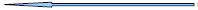Outer for( ) loop
{
for( int j = 1 ; j<=3 ; j++)Inner for( ) loop
{
cout<< i * j <<endl ;
}
}

For each iteration of the outer for loop the inner for loop will iterate fully up to the last value of inner loop iterator. The situation can be understood more clearly as :

1st Outer Iteration
i= 1
1st Inner Iteration
j = 1 , output : 1 * 1 = 1
2nd Inner Iteration
j = 2 , output : 1 * 2 = 2
3rd Inner Iteration
j = 3 , output : 1 * 3 = 3

2nd Outer Iteration
i= 2
1st Inner Iteration
j = 1 , output : 2 * 1 = 1
2nd Inner Iteration
j = 2 , output : 2 * 2 = 4
3rd Inner Iteration
j = 3 , output : 2 * 3 = 6

You can observe that j is iterated from 1 to 2 every time i is iterated once.

##### Jump Statements:-

These statements unconditionally transfer control within function . In C++ four statements perform an unconditional branch :
1. return
2. goto
3. break
4. continue

1. return Statement:- The return statement is used to return from a function. It is useful in two ways:
(i) An immediate exit from the function and the control passes back to the operating system
which is main’s caller.
(ii) It is used to return a value to the calling code.

2. goto statement :- A goto Statement can transfer the program control anywhere in the program. The target destination of a goto statement is marked by a label. The target label and goto must appear in the same function. The syntax of goto statement is:

goto label;
label :
Example :
a= 0;
start :
cout<<“\n” <<++a;
if(a<50) goto start;

###### 3. break Statement :-

The break statement enables a program to skip over part of the code. A break statement terminates the smallest enclosing while, do-while, for or switch statement. Execution resumes at the statement immediately following the body of the terminated statement. The following figure explains the working of break statement: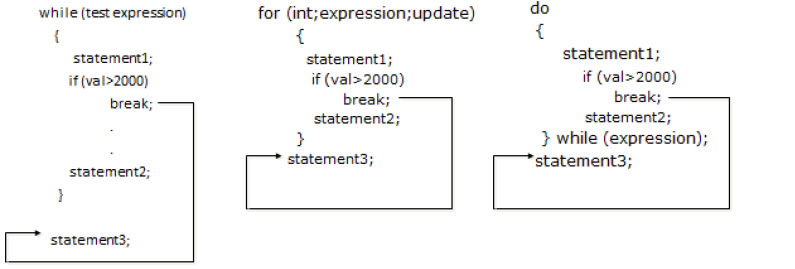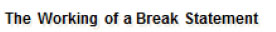###### 4. continue Statement:-

The continue is another jump statement like the break statement as both the statements skip over a part of the code. But the continue statement is somewhat different from break. Instead of forcing termination, it forces the next iteration of the loop to take place, skipping any code in between. The following figure explains the working of continue statement: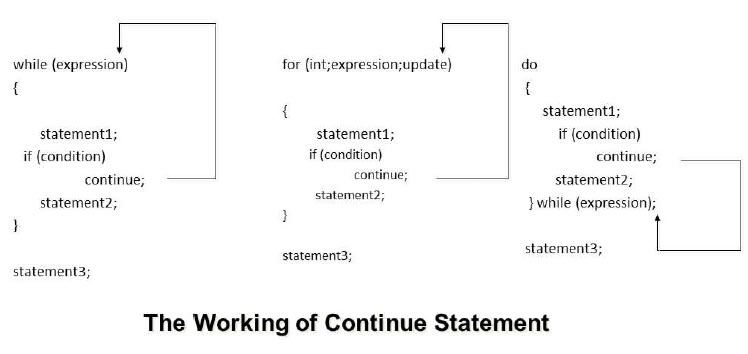###### Functions :-

Function is a named group of programming statements which perform a specific task and return a value.
There are two types of functions:-
1. Built-in (Library )functions
2. User defined functions

###### Built-in Functions (Library Functions) :-

The functions, which are already defined in C++ Library ( in any header files) and a user can directly use these function without giving their definition is known as built-in or library functions. e.g., sqrt( ), toupper( ), isdigit( ) etc. Following are some important Header files and useful functions within them :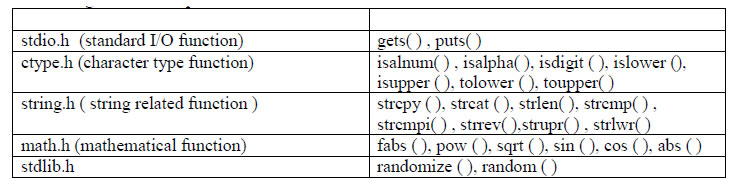The above list is just few of the header files and functions available under them , but actually there are many more. The calling of library function is just like User defined function , with just few differences as follows:
i) We don't have to declare and define library function.
ii) We must include the appropriate header files , which the function belongs to, in global area so as these functions could be linked with the program and called.
Library functions also may or may not return values. If it is returning some values then the value should be assigned to appropriate variable with valid datatype.

gets( ) and puts( ) : these functions are used to input and output strings on the console during program run-time.

gets( ) accept a string input from user to be stored in a character array.
puts() displays a string output to user stored in a character array.
Program: Program to use gets( ) and puts( )
#include<iostream.h>
#include<stdio.h> // must include this line so that gets( ) , puts( ) could be linked and
// called
void main( )
{
char myname ; //declaring a character array of size 25
gets(myname) ; // just pass the array name into the parameter of the function.
cout<< “You have inputted your name as : “ ;
puts(myname);
}

isalnum( ) , isalpha( ), isdigit( ) : checks whether the character which is passed as parameter to them are alphanumeric or alphabetic or a digit ('0' to '9') . If checking is true functions returns 1.

Program : Program to use isalnum( ) , isalpha( ), isdigit( )
#include<iostream.h>
#include<ctype.h>
void main( )
{
char ch;
cout<<”Input a character”;
cin>>ch;
if( isdigit(ch) = = 1)
cout<<”The inputed character is a digit”;
else if(isalnum(ch) = = 1)
cout<<”The inputed character is an alphanumeric”;
else if(isalpha(ch) = = 1)
cout<<”The inputed character is an alphabet.
}

islower ( ),isupper ( ), tolower ( ), toupper( ) : islower( ) checks whether a character is lower case , isupper( ) check whether a character is upper case . tolower( ) converts any character passed to it in its lower case and the toupper( ) convert into upper case.

Program: Program to use islower ( ),isupper ( ), tolower ( ), toupper( )

#include<iostream.h>
#include<ctype.h>
void main( )
{
char ch;
cout<<”Input a character”;
cin>>ch;
if( isupper(ch) = = 1) // checks if character is in upper case converts the character
// to lower case
{
tolower(ch);
cout<<ch;
}
else if(islower(ch) = = 1) // checks if character is in lower case converts the
// character to uppercase
{
toupper(ch);
cout<<ch;
}
}

fabs ( ), pow ( ), sqrt ( ), sin ( ), cos ( ), abs ( ) :

Program 3.4
#include <iostream.h>
#include <math.h>
#define PI 3.14159265 // macro definition PI will always hold 3.14159265
void main ()
{
cout<<"The absolute value of 3.1416 is : “<<fabs (3.1416) ;
// abs( ) also acts similarly but only on int data
cout<<"The absolute value of -10.6 is "<< fabs (-10.6) ;
cout<<”7.0 ^ 3 = " <<pow (7.0,3);
cout<<"4.73 ^ 12 = " <<pow (4.73,12);
cout<<"32.01 ^ 1.54 = "<<pow (32.01,1.54);
double param, result;
param = 1024.0;
result = sqrt (param);
cout<<"sqrt() = "<<result ;
result = sin (param*PI/180); // in similar way cos( ) , tan() will be called.
cout<<"The sine of “ <<param<<” degrees is : "<< result
}

randomize ( ), random ( ) : The above functions belongs to header file stdlib.h . Let us observe the use of these functions :

randomize( ) : This function provides the seed value and an algorithm to help random( ) function in generating random numbers. The seed value may be taken from current system’s time.

random( ) : This function accepts an integer parameter say x and then generates a random value between 0 to x-1 for example : random(7) will generate numbers between 0 to 6.

To generate random numbers between a lower and upper limit we can use following formula random(U – L +1 ) + L where U and L are the Upper limit and Lower limit values between which we want to find out random values.
For example : If we want to find random numbers between 10 to 100 then we have to write code as :

random(100 -10 +1) + 10 ; // generates random number between 10 to 100

###### User-defined function :-

The functions which are defined by user for a specific purpose is known as user-defined function. For using a user-defined function it is required, first define it and then using. Function

###### Prototype :-

Each user define function needs to be declared before its usage in the program. This declaration is called as function prototype or function declaration. Function prototype is a declaration statement in the program and is of the following form :
Return_type function_name(List of formal parameters) ;

###### Declaration of user-defined Function:

In C++ , a function must be defined, the general form of a function definition is :
Return_type function_name(List of formal arameters)

{
Body of the function
}

Where Return_type is the data type of value return by the function. If the function does not return any value then void keyword is used as return_type. List of formal parameters is a list of arguments to be passed to the function. Arguments have data type followed by identifier. Commas are used to separate different arguments in this list. A function may be without any parameters, in which case , the parameter list is empty. statements is the function's body. It is a block of statements surrounded by braces { }. Function_name is the identifier by which it will be possible to call the function.

e.g.,
int addition (int a, int b)
{
int r ;
r=a+b ;
return (r) ;
}

Calling a Function:- When a function is called then a list of actual parameters is supplied that should match with formal parameter list in number, type and order of arguments. Syntax for calling a function is:
function_name ( list of actual parameters );

e.g.,
#include <iostream>
int addition (int a, int b)
{ int r;
r=a+b;
return (r); }
void main ( )
{ int z ;
cout<< "The result is " << z;
}
The result is 8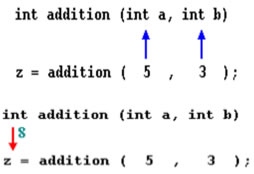##### Call by Value (Passing by value) :-

The call by value method of passing arguments to a function copies the value of actual parameters into the formal parameters , that is, the function creates its own copy of argument values and then use them, hence any chance made in the parameters in function will not reflect on actual parameters . The above given program is an example of call by value.

###### Call by Reference ( Passing by Reference) :-

The call by reference method uses a different mechanism. In place of passing value to the function being called , a reference to the original variable is passed . This means that in call by reference method, the called function does not create its own copy of original values , rather, its refers to the original values only by different names i.e., reference . thus the called function works the original data and any changes are reflected to the original values.

// passing parameters by reference
#include <iostream.h>
void duplicate (int& a, int& b, int& c)
{
a*=2;
b*=2;
c*=2;
}
void main ()
{
int x=1, y=3, z=7;
duplicate (x, y, z);
cout <<"x="<< x <<", y="<< y <<", z="<< z;
}
output :x=2, y=6, z=14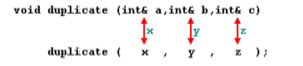The ampersand (&) (address of) is specifies that their corresponding arguments are to be passed by reference instead of by value.

###### Constant Arguments:-

In C++ the value of constant argument cannot be changed by the function. To make an argument constant to a function , we can use the keyword const as shown below: 54 int myFunction( const int x , const int b ); The qualifier const tell the compiler that the function should not modify the argument. The compiler will generate an error when this condition is violated.

###### Default Arguments :-

C++ allows us to assign default value(s) to a function’s parameter(s) which is useful in case a matching argument is not passed in the function call statement. The default values are specified at the time of function definition. e.g., float interest ( float principal, int time, float rate = 0.70f)

Here if we call this function as:
si_int= interest(5600,4);
then rate =0.7 will be used in function.

###### Formal Parameters:-

The parameters that appear in function definition are formal parameters. Actual

###### Parameters :-

The parameters that appears in a function call statement are actual parameters. Functions with no return type (The use of void):- If you remember the syntax of a function declaration:
Return_type function_name(List of formal parameters)
you will see that the declaration begins with a type, that is the type of the function itself (i.e., the data type of value that will be returned by the function with the return statement). But what if we want to return no value? Imagine that we want to make a function just to show a message on the screen. We do not need it to return any value. In this case we should use the void type specifier for the function. This is a special specifier that indicates absence of type.

###### The return Statement :-

The execution of return statement, it immediately exit from the function and control passes back to the calling function ( or, in case of the main( ), transfer control back to the operating system). The return statement also returns a value to the calling function. The syntax of return statement is: return ( value);

###### Scope of Identifier :-

The part of program in which an identifier can be accessed is known as scope of that identifier. There are four kinds of scopes in C++
(i) Local Scope :- An identifier declare in a block ( { } ) is local to that block and can be used only in it.
(ii) Function Scope :- The identifier declare in the outermost block of a function have function scope.
(iii) File Scope ( Global Scope) :- An identifier has file scope or global scope if it is declared outside all blocks i.e., it can be used in all blocks and functions.
(iv) Class Scope :- A name of the class member has class scope and is local to its class.

The time interval for which a particular identifier or data value lives in the memory is called Lifetime of the identifier or data value.

### NCERT Books Free Pdf Download for Class 5, 6, 7, 8, 9, 10 , 11, 12 Hindi and English Medium

 Mathematics Biology Psychology Chemistry English Economics Sociology Hindi Business Studies Geography Science Political Science Statistics Physics Accountancy